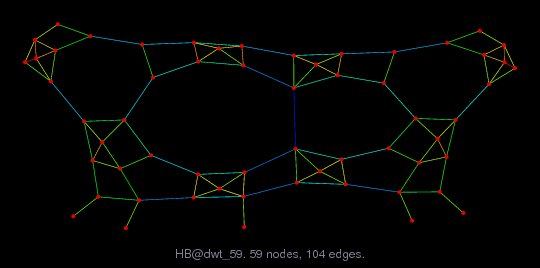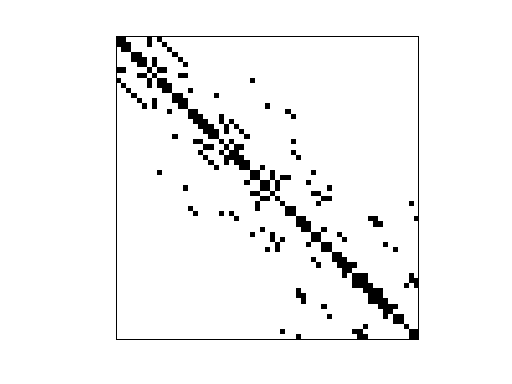Matrix: HB/dwt_59

Description: SYMMETRIC CONNECTION TABLE FROM DTNSRDC, WASHINGTON(undirected graph drawing)• Matrix group: HB
• download as a MATLAB mat-file, file size: 899 bytes. Use UFget(129) or UFget('HB/dwt_59') in MATLAB.

 Matrix properties number of rows 59 number of columns 59 nonzeros 267 structural full rank? yes structural rank 59 # of blocks from dmperm 1 # strongly connected comp. 1 explicit zero entries 0 nonzero pattern symmetry symmetric numeric value symmetry symmetric type binary structure symmetric Cholesky candidate? yes positive definite? no

 author G. Everstine, D. Taylor editor I. Duff, R. Grimes, J. Lewis date 1980 kind structural problem 2D/3D problem? yes

 Ordering statistics: result nnz(chol(P*(A+A'+s*I)*P')) with AMD 244 Cholesky flop count 1.1e+03 nnz(L+U), no partial pivoting, with AMD 429 nnz(V) for QR, upper bound nnz(L) for LU, with COLAMD 271 nnz(R) for QR, upper bound nnz(U) for LU, with COLAMD 506

 SVD-based statistics: norm(A) 4.96642 min(svd(A)) 0.0420518 cond(A) 118.102 rank(A) 59 sprank(A)-rank(A) 0 null space dimension 0 full numerical rank? yes

 singular values (MAT file): click here SVD method used: s = svd (full (A)) ; status: ok